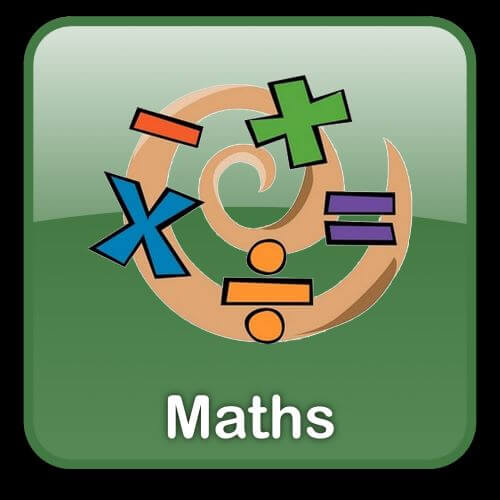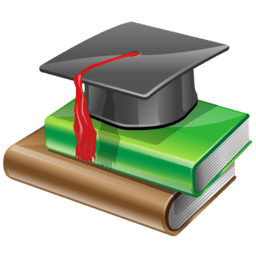## Secondary 3 Maths# Maths Tuition Centre for Secondary class 3.

Students in the Maths Tuition Centre for secondary class three will get exposure to additional mathematics. This subject can get a little tough for students with the introduction of new lengthy and tough formulas but with proper guidance and education one can learn them very easily. Students will be taught how to solve such complex problems using the right techniques.

About the course- This course will teach students various topics mentioned below-

• Students are taught topics such as equation and inequality, indices and surds, binomial expansions and polynomial and partial fractions.
• Students will be taught the relationship of sine and cosine to science along with propagation of sound waves.
• Students will be taught how to find equation of circles, and the area and perimeter of the rectilinear figure in a coordinate plane.
• Students will be taught how to prove that two trigonometrical expressions are identical using identities and formulas.

Target students – This course is meant only students of secondary class three both normal and express academic, who want to learn the best mathematics tricks and techniques required to solve problems.

Schedule: Weekdays (Monday to Friday: 5.30 pm to 8.30 pm) & Saturday and Sunday: 9.30 am to 4 pm.

Venue: 10 Anson Road, 26-08A International Plaza, Singapore 079903

##Secondary School Mathematics (Syllabus)

• Proportions

### Mensuration

• Volume and Surface Area of Solids

### Algebra

• Linear Graphs and Simultaneous Linear Equations
• Expansion of Algebraic Expressions
• Factorisation of Algebraic Expressions
• Quadratic Equations and Graphs
• Algebraic Fractions and Manipulations

### Geometry

• Congruency and Similarity
• Maps and Scales
• Trigonometry
• Pythagoras Theorem
• Trigonometric Ratios and Applications

• Probability

### Statistics

• Data Handling
• Data Analysis
• Data Analysis of Grouped Data

### Equation and Inequalities

1.1. Simultaneous Equations
1.1.1. Solve a pair of simultaneous linear and non-linear equations
1.1.2. Find the point(s) of intersection of two graphs algebraically
1.1.3. Formulate and solve simultaneous equations to model real-world
problems
1.2. Sum and Product of Roots
1.2.1. Use the formulae for the sum and product of roots of a quadratic
equation
1.2.2. Form a quadratic equation from its roots
1.3. Discriminant, Roots and x-intercepts
1.3.1. Sketch quadratic graphs
1.3.2. Use discriminant to determine the nature of roots
1.3.3. Use discriminant to determine the number of points of intersection of
two graphs.
1.4. Quadratic Inequalities
1.4.1. Solve quadratic inequalities graphically
1.4.2. Solve inequality problems involving the discriminant, roots and x-
intercepts
1.4.3. Solve simultaneous inequalities

### Surds and Indices

2.1. Surds
2.1.1. Simplify surds
2.1.2. Rationalise fractions with surds in their denominators
2.1.3. Solve equations involving surds
2.2. Indices
2.2.1. Simplify expressions using the definition and properties of indices
2.2.2. Evaluate expressions involving indices and surds to find unknowns
2.3. Equations Involving Indices
2.3.1. Use the property (ax=ay x = n) to solve equations involving indices
2.3.2. Use substitution to solve equations involving indices
2.4. Exponential Functions
2.5. Sketch graphs of exponential functions
2.6. Use exponential functions in real-world contexts

### Polynomials and partial fraction

3.1. Polynomial and identities
3.1.1. Identify polynomials
3.1.2. Add and subtract polynomials
3.1.3. Multiply polynomials
3.1.4. Find the unknowns in an identity
3.2. Division of Polynomials
3.2.1. Divide two polynomials
3.2.2. Express the result of division in terms of quotient, divisor and
remainder
3.3. The Remainder Theorem
3.3.1. Use the remainder theorem to find remainder
3.3.2. Use the remainder theorem to find unknown coefficients or find the
relationship between unknown coefficients.
3.4. The Factor Theorem
3.4.1. Apply the factor theorem to find factors of a polynomial
3.4.2. Apply the factor theorem to find unknown coefficients or the
relationship between unknown coefficients
3.4.3. Factorise expressions of the form a 2 +b 2 and a 2 – b 2
3.5. Cubic Polynomials and Equations
3.5.1. Factorise or determine factors of cubic expressions
3.5.2. Solve cubic expressions
3.6. Partial Fractions
3.6.1. Express a proper algebraic fraction into its partial fractions
3.6.2. Identify whether an algebraic fraction is a proper or an improper
fraction
3.6.3. Express an improper algebraic fraction in partial fractions

### The Modulus and Power Functions

4.1. Modulus Functions and Their Graphs
4.1.1. Simplify and evaluate modulus expressions
4.1.2. Solve equations involving modulus
4.1.3. Sketch graphs of y=If(x)l
4.2. Graph of Power Functions
4.2.1. Sketch graphs of power functions
4.2.2. Solve problems on intersections of curves with one power graph

### Binomial Theorem

5.1. The Binomial Expansion of (1+b) n
5.1.1. Use the notations n! and ( )
5.1.2. Expand (1+b) n where n is a positive integer
5.2. The Binomial Expansion of (a+b) n
5.2.1. Expand (a+b) n
5.2.2. Use the general term formula to find specific terms, coefficients and unknown values.

### Points Lines and Shapes

6.1. Mid-point of a line segment
6.1.1. Use the mid-point formula
6.1.2. Apply the mid-point formula to solve geometric problems
6.2. Parallel Lines
6.2.1. Relate gradient to the angle of inclination
6.2.2. Find hoe the gradients of two parallel lines are related
6.2.3. Find the equation of a line that is parallel to a given line
6.3. Perpendicular Lines
6.3.1. Find how the gradients of two perpendicular lines are related
6.3.2. Find the equation of a line that is perpendicular to a given line
6.3.3. Find the equation of a perpendicular bisector of a line segment
6.4. Areas of Triangles and Quadrilaterals
6.4.1. Use the formulae for the area of a triangle
6.4.2. Use the formulae for the area of a quadrilateral

### Logarithms

7.1. Introduction to Logarithms
7.1.1. State what a logarithm is and use logarithmic notations
7.1.2. Use the equivalence of y=ax and x=logaY
7.1.3. Evaluate special logarithmic values
7.1.4. Apply the concept of logarithm to solve equations
7.2. Laws of logarithms
7.2.1. Use the power, product and quotient laws to manipulate logarithms
7.2.2. Use the change-of-base law to manipulate logarithms
7.3. Logarithmic Equations
7.3.1. Apply the law for the same base to solve logarithmic equations
7.3.2. Apply the change-of-base law to solve equations
7.4. Logarithms and Equations of the Form a x =b
7.4.1. Use logarithms to solve equations of the for, ax=b
7.5. Graphs and Applications of Logarithmic Function
7.5.1. Draw graphs of logarithmic functions
7.5.2. Solve real-world problems on logarithmic functions

### Application of Straight Lines Graphs

8.1. Reducing Equations to Linear Form
8.1.1. Reduce equations to linear form and plot straight line graphs
8.1.2. Obtain non-linear equations from straight line equations or graphs
8.2. Linear Laws
8.2.1. Sketch and interpret lines of closest fit
8.2.2. Solve real-world problems on linear law

### Parabola and Circles

9.1. Graph of Parabolas of the Formy 2 =kx
9.1.1. Sketch parabolic graphs of the form y2=kx
9.1.2. Solve geometric problems involving the intersection of lines and curves
9.2. Coordinates Geometry of Circles
9.2.1. Find the equation, the center and the radius of a circle
9.2.2. Solve geometric problems involving the intersection of lines and circles.

##Why Choose Inspigenius?

Students here at inspigenius are provided an enriching atmosphere to study along with the following mentioned benefits-

• Students are taught in small batch classes that help them understand better whatever is being taught.
• Students are taught by experts in their respective field that provide the best education along with other skills such as time management during the examination.
• Students are given quality study material that they can refer to at all times for study related queries.
• Regular assessment take place that help study to catch hold of his weak points really quick and work on them.